# Forces and Motion JEOPARDY Force Motion Vector Laws

• Slides: 54Forces and MotionJEOPARDY Force Motion Vector Laws Gravity 100 100 100 200 200 200 300 300 300 400 400 400 500 500 500 Final Jeopardy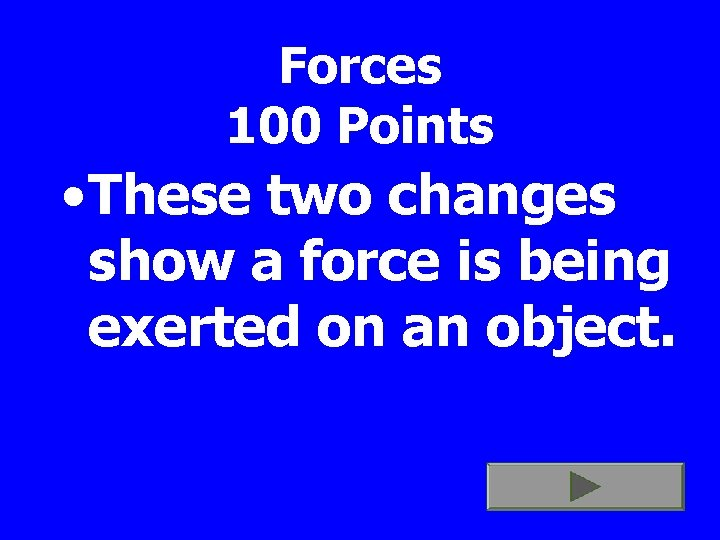Forces 100 Points • These two changes show a force is being exerted on an object.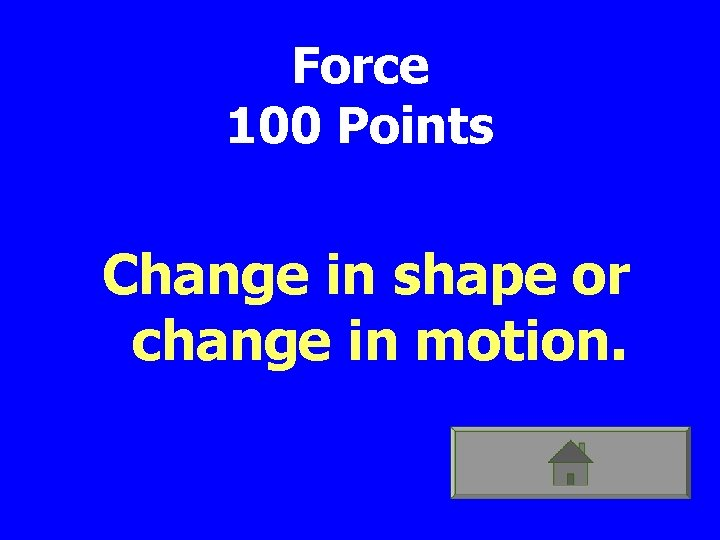Force 100 Points Change in shape or change in motion.Force 200 Points • A force is either one of these two things.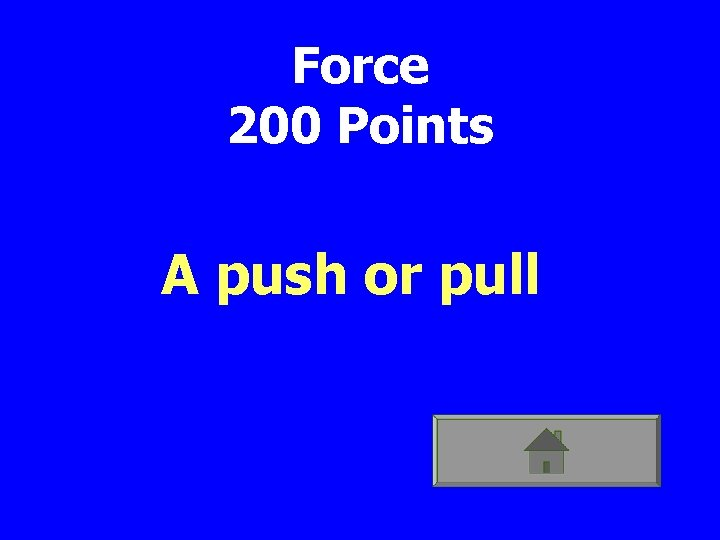Force 200 Points A push or pullForce 300 Points • The direction the people will travel. 250 N 350 N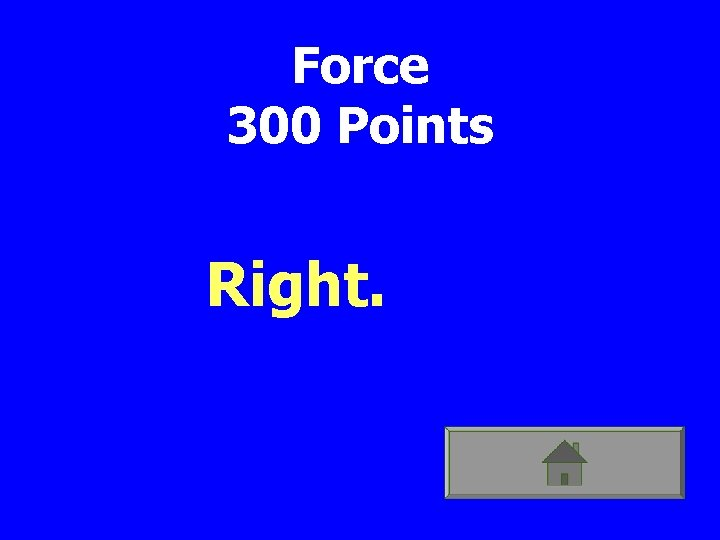Force 300 Points Right.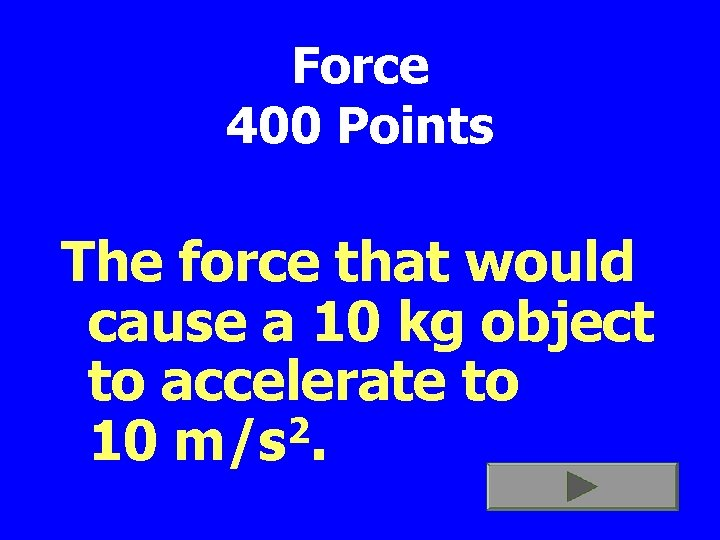Force 400 Points The force that would cause a 10 kg object to accelerate to 2 10 m/s.Force 400 Points 100 NForce 500 Points The formula for calculating how much force is acting on an object.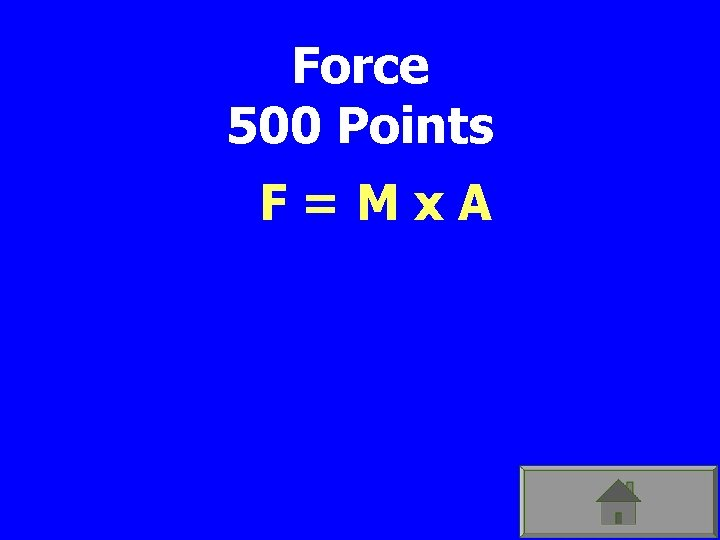Force 500 Points F=Mx. A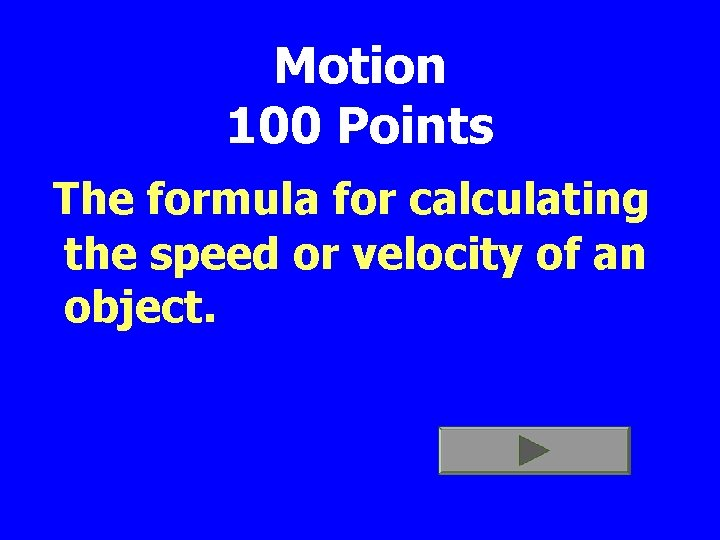Motion 100 Points The formula for calculating the speed or velocity of an object.Motion 100 Points S = D/T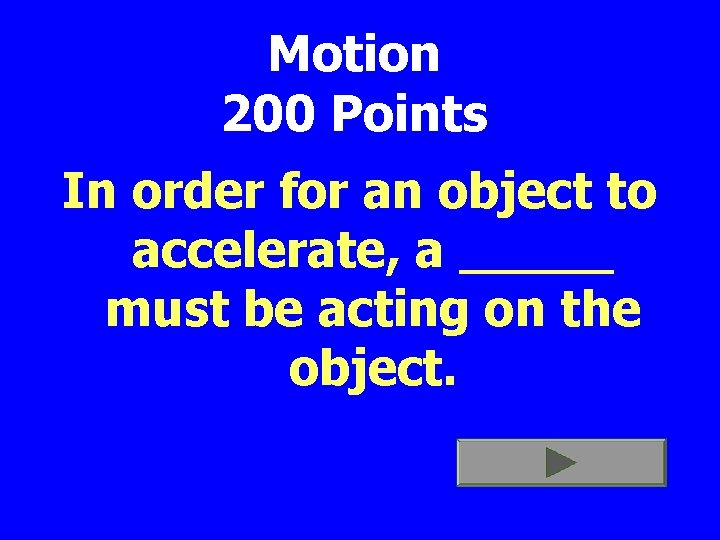Motion 200 Points In order for an object to accelerate, a _____ must be acting on the object.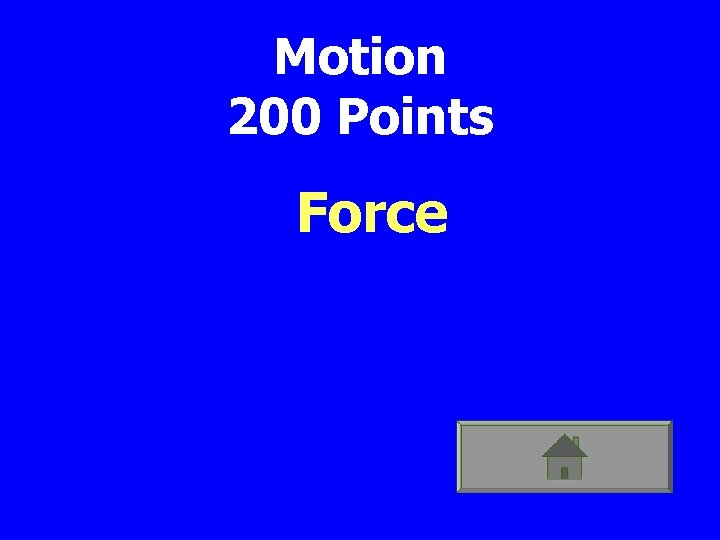Motion 200 Points ForceMotion 300 Points This describes both speed and direction.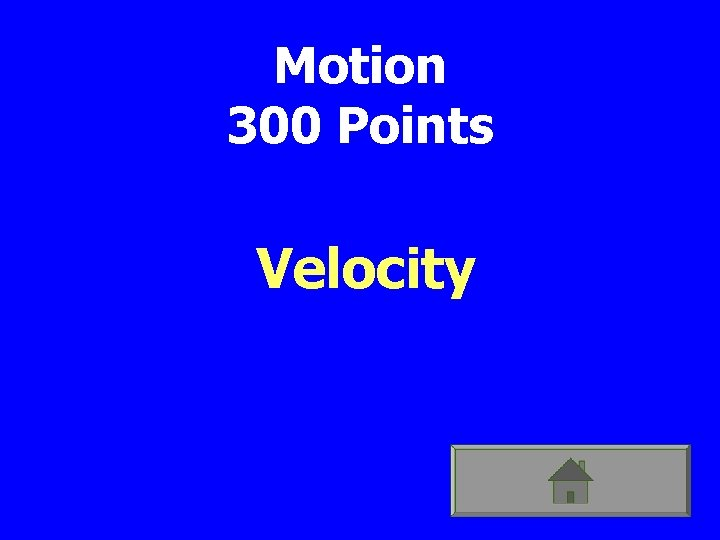Motion 300 Points VelocityMotion 400 Points Your frame of reference when you are in this classroom.Motion 400 Points The classroom.Motion 500 points Any change in speed or direction.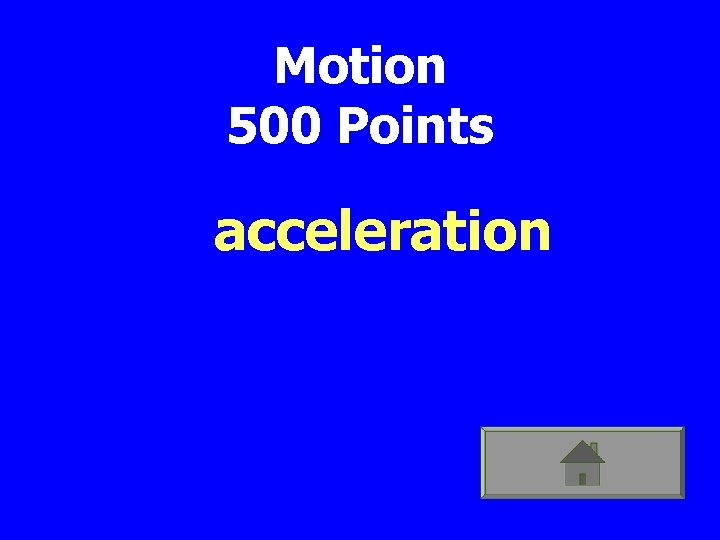Motion 500 Points acceleration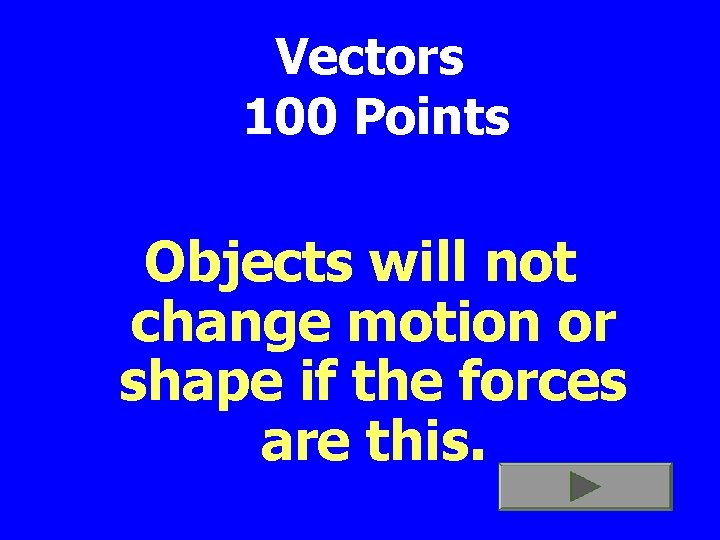Vectors 100 Points Objects will not change motion or shape if the forces are this.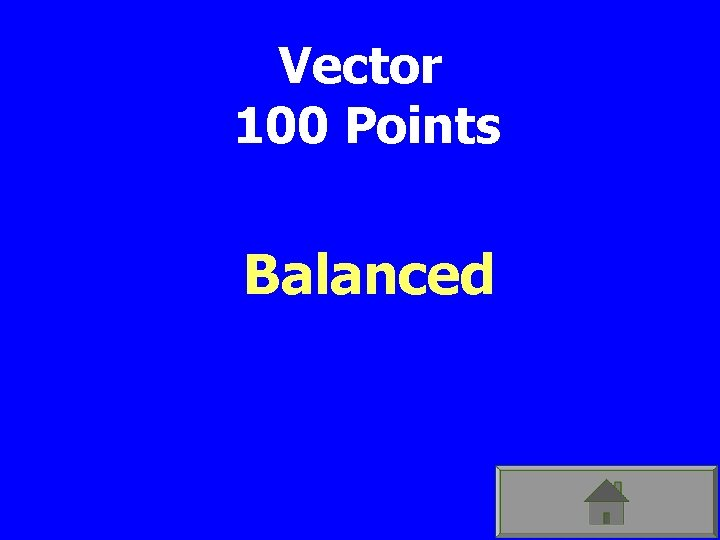Vector 100 Points BalancedVector 200 Points How much friction is represented in the diagram? 10 N Mass =50 Kg 75 N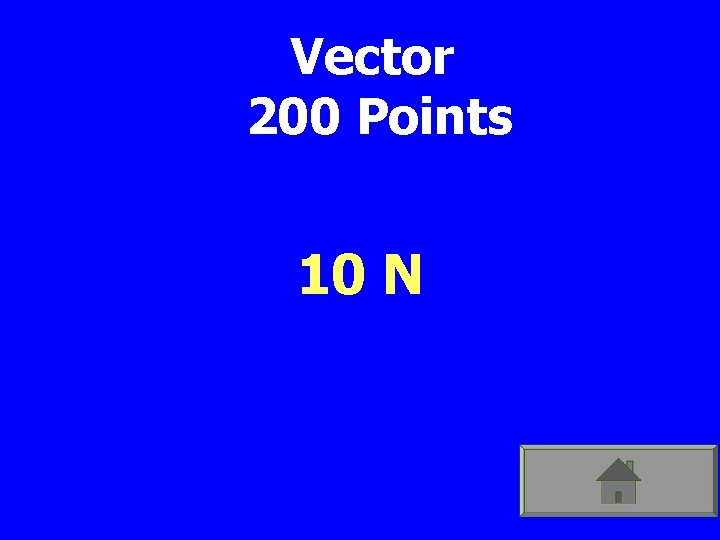Vector 200 Points 10 NVector 300 Points Which direction will the cart move? 10 N Mass =50 Kg 75 NVector 300 Points LeftVector 400 Points What is the force needed to have the object travel at a constant velocity? 500 N ?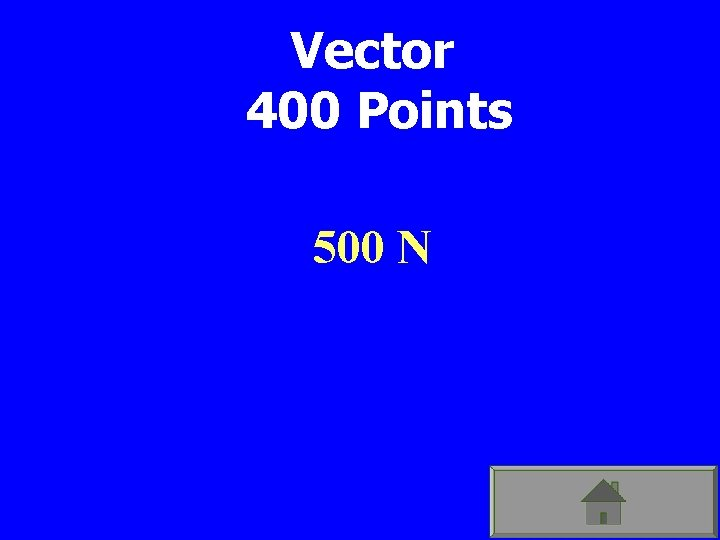Vector 400 Points 500 N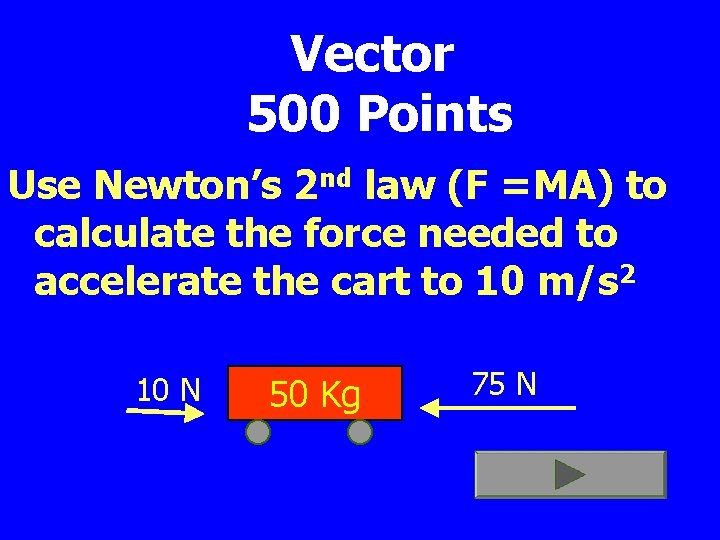Vector 500 Points Use Newton’s 2 nd law (F =MA) to calculate the force needed to accelerate the cart to 10 m/s 2 10 N 50 Kg 75 NVector 500 Points 500 Newtons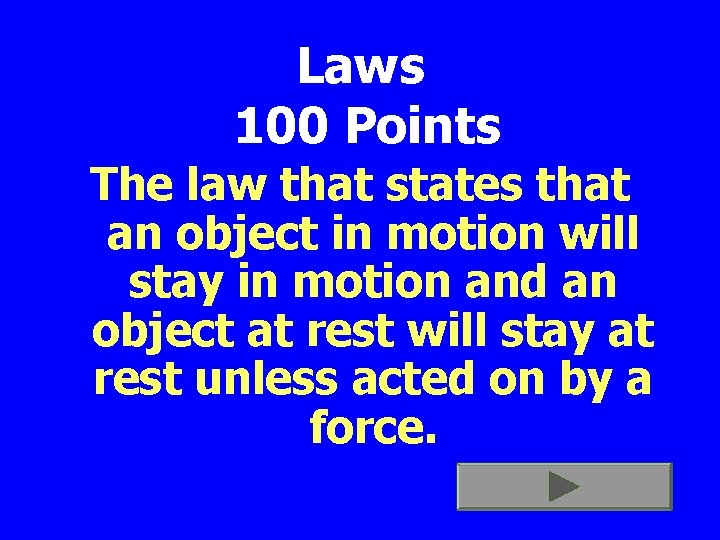Laws 100 Points The law that states that an object in motion will stay in motion and an object at rest will stay at rest unless acted on by a force.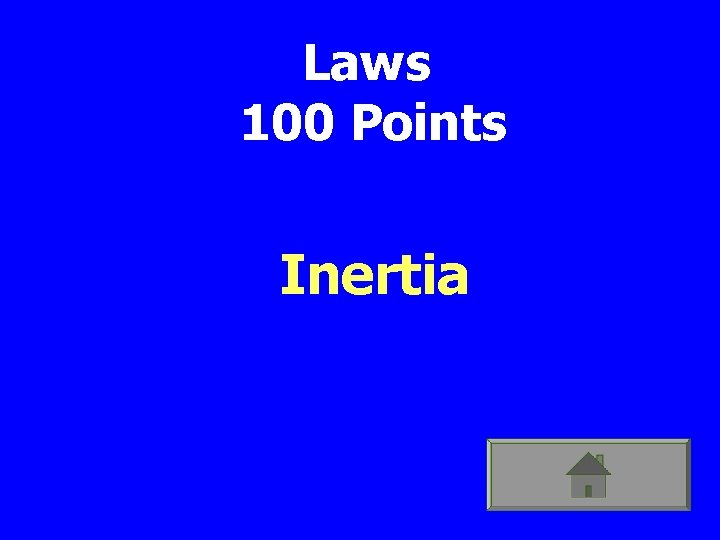Laws 100 Points Inertia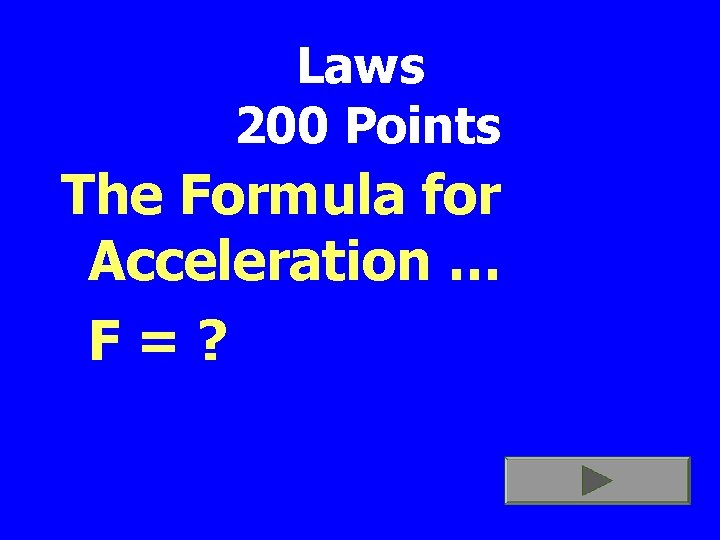Laws 200 Points The Formula for Acceleration … F=?Laws 200 Points F=Mx. A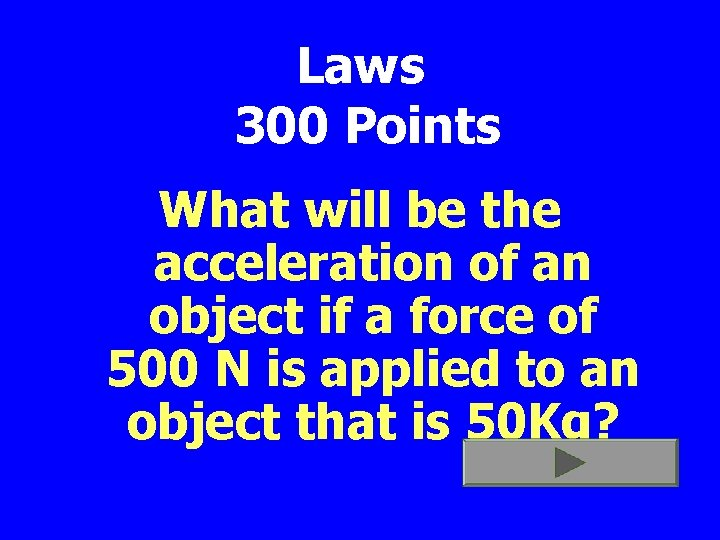Laws 300 Points What will be the acceleration of an object if a force of 500 N is applied to an object that is 50 Kg?Laws 300 Points 10 2 m/secLaws 400 Points The law that allows a rocket to be launched up if its exhaust is pushing down.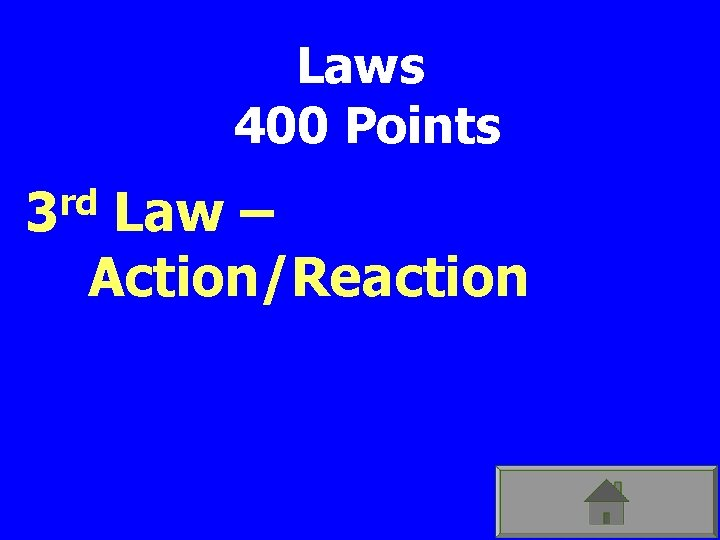Laws 400 Points rd 3 Law – Action/ReactionLaws 500 Points rd 3 The Law states that …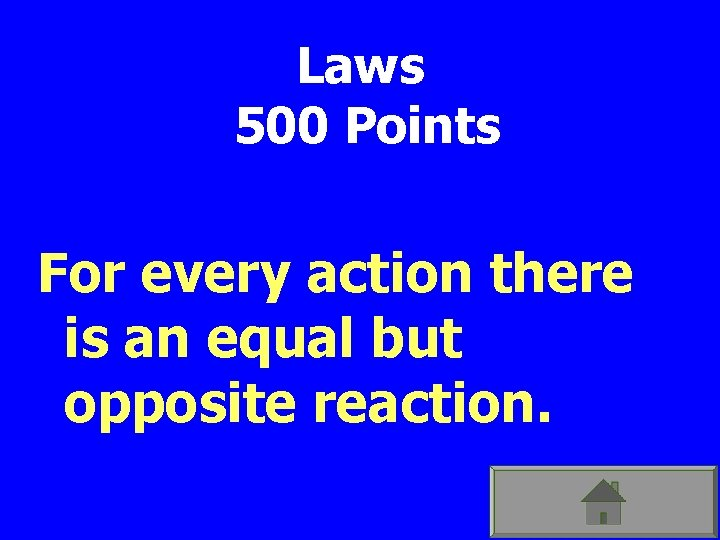Laws 500 Points For every action there is an equal but opposite reaction.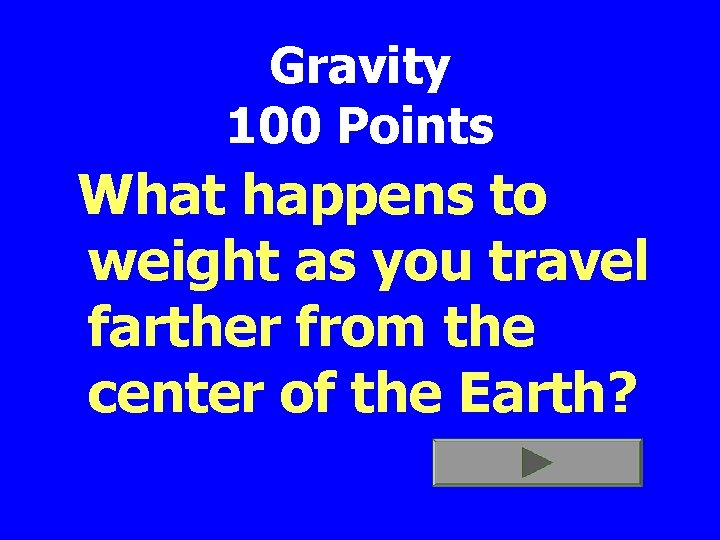Gravity 100 Points What happens to weight as you travel farther from the center of the Earth?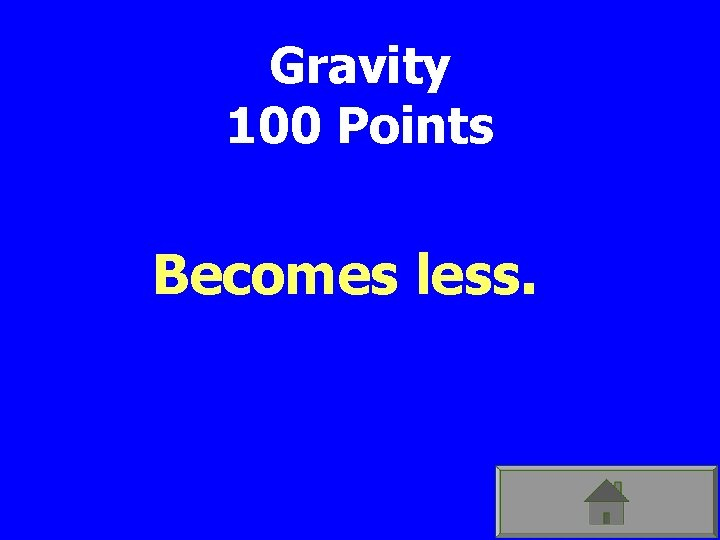Gravity 100 Points Becomes less.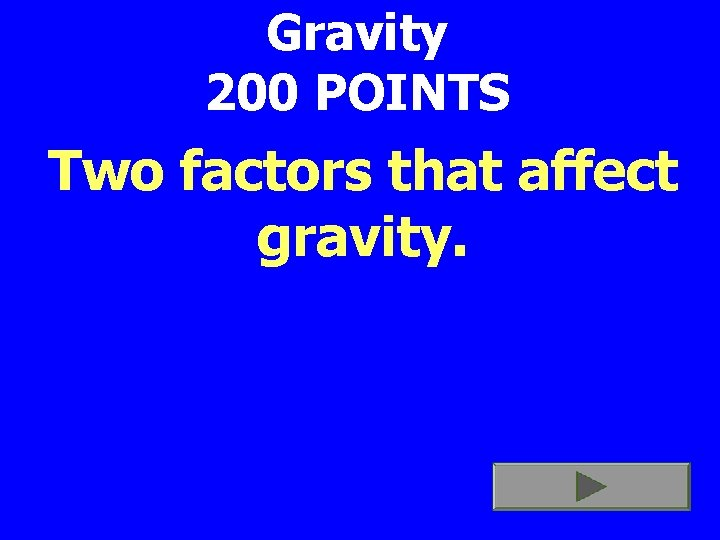Gravity 200 POINTS Two factors that affect gravity.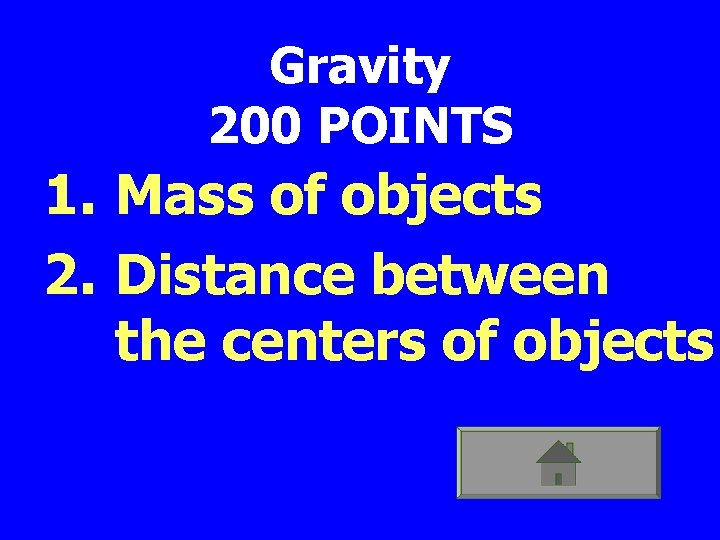Gravity 200 POINTS 1. Mass of objects 2. Distance between the centers of objects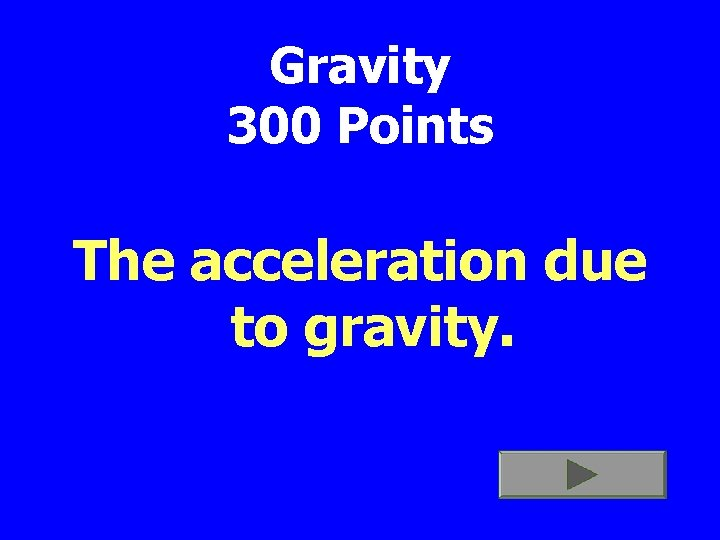Gravity 300 Points The acceleration due to gravity.Gravity 300 Points 9. 8 2 m/sec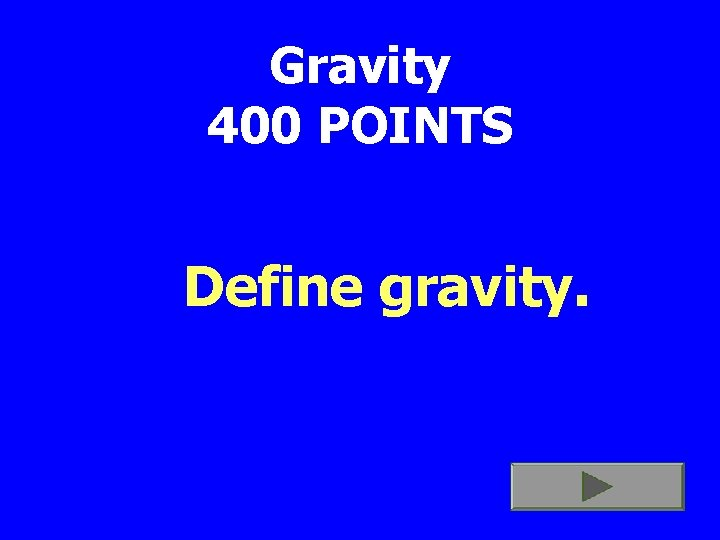Gravity 400 POINTS Define gravity.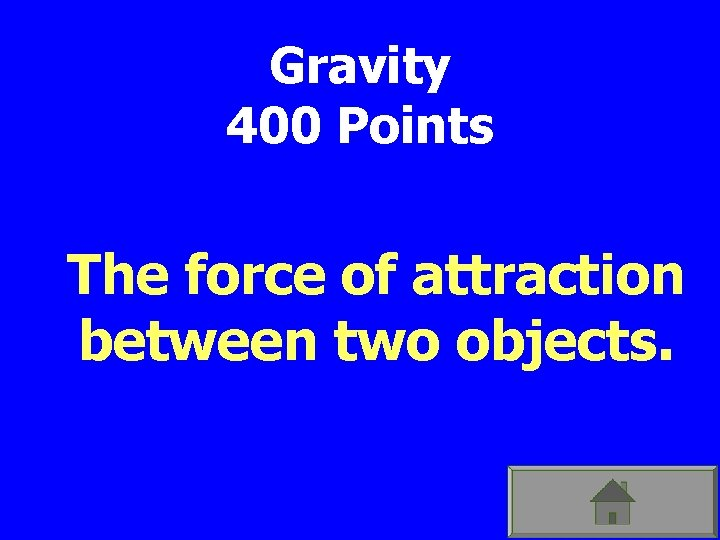Gravity 400 Points The force of attraction between two objects.Gravity 500 POINTS • The acceleration of an object that has been falling for 3 seconds.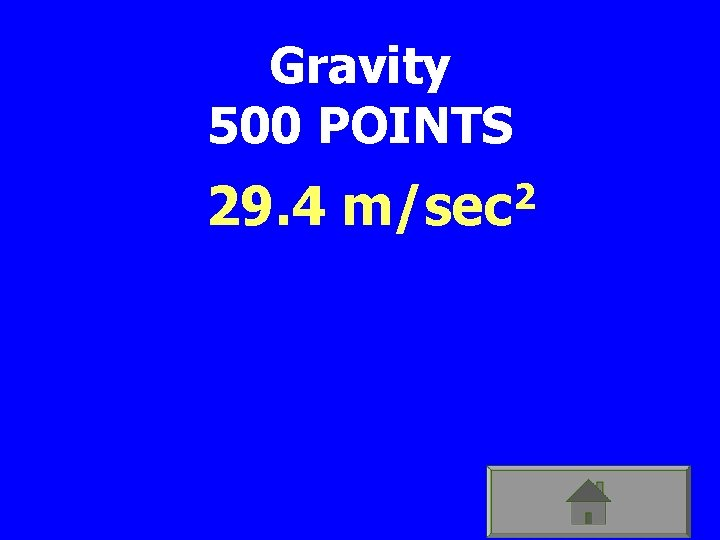Gravity 500 POINTS 29. 4 2 m/secFINAL JEOPARDY Objects that are traveling, will travel in this type of path unless acted on by a force.FINAL JEOPARDY Straight Path